Galvanic cells (2020 VCE) 1 A diagram of an electrochemical cell is shown below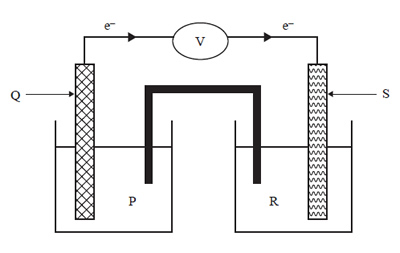Which of the following gives the correct combination of the electrode in the oxidation half-cell and the electrolyte in the reduction half-cell?.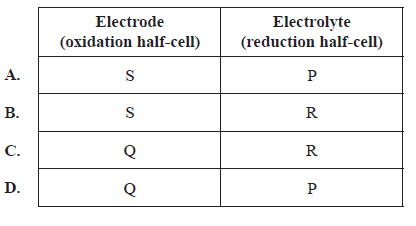Solution2) Which one of the following pairs of statements is correct for both electrolysis cells and galvanic cells?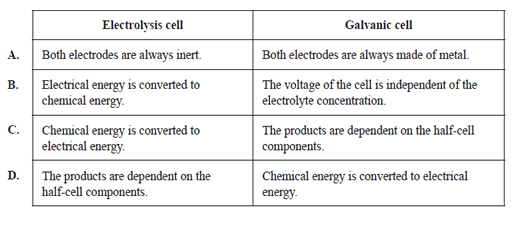Solution3) The following reactions occur in a primary cell battery.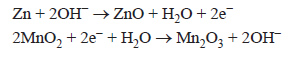Which one of the following statements about the battery is correct? A. The reaction produces heat and Zn reacts directly with MnO2. B. The reaction produces heat and Zn does not react directly with MnO2. C. The reaction does not produce heat and Zn reacts directly with MnO2. D. The reaction does not produce heat and Zn does not react directly with MnO2. Solution4) Consider the following half-equation.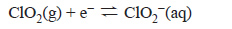It is also known that: • ClO2(g) will oxidise HI(aq), but not HCl(aq) • Fe3+(aq) will oxidise HI(aq), but not NaClO2(aq). Based on this information, Fe2+(aq) can be oxidised by A. Cl2(g) and I2(aq). B. Cl2(g), but not ClO2(g). C. ClO2(g) and Cl2(g), but not I2(aq). D. Cl2(g), ClO2(g) and I2(aq). Solution5) Research scientists are developing a rechargeable lithium–carbon dioxide, Li–CO2, battery. The rechargeable Li–CO2 battery is made of lithium metal, carbon in the form of graphite (coated with a catalyst) and a non-aqueous electrolyte that absorbs CO2. A diagram of the rechargeable Li–CO2 cell is shown below. One Li–CO2 cell generates 4.5 V.When the Li–CO2 cell generates electricity, the two half-cell reactions are 4Li+ + 3CO2 + 4e− → 2Li2CO3+ C Li → Li+ + e− a) Write the equation for the overall recharge reaction. Solutionb) During discharge, lithium carbonate, Li2CO3, deposits break away from the electrode. Describe how this might affect the performance of the battery. Solutionc) Explain why it is unsafe to use an aqueous electrolyte in the design of the Li–CO2 battery. Include appropriate equations in your answer. Solutiond. Could the Li–CO2 battery be used to reduce the amount of CO2(g) in the atmosphere? Give your reasoning. Solution